The OpenFOAM Foundation
solidMixtureProperties Class Reference

A mixture of solids. More...

## Public Member Functions

solidMixtureProperties (const dictionary &)
Construct from dictionary. More...

solidMixtureProperties (const solidMixtureProperties &lm)
Construct copy. More...

virtual autoPtr< solidMixturePropertiesclone () const
Construct and return a clone. More...

virtual ~solidMixtureProperties ()
Destructor. More...

const List< word > & components () const
Return the solidProperties names. More...

const PtrList< solidProperties > & properties () const
Return the solidProperties properties. More...

label size () const
Return the number of solids in the mixture. More...

scalarField Y (const scalarField &X) const
Returns the mass fractions, given mole fractions. More...

scalarField X (const scalarField &Y) const
Returns the mole fractions, given mass fractions. More...

scalar rho (const scalarField &X) const
Calculate the mixture density [kg/m^3] as a function of. More...

scalar Cp (const scalarField &Y) const
Calculate the mixture heat capacity [J/(kg K)] as a function. More...

## Static Public Member Functions

static autoPtr< solidMixturePropertiesNew (const dictionary &)
Select construct from dictionary. More...

## Detailed Description

A mixture of solids.

An example of a two component solid mixture:

```    <parentDictionary>
{
C
{
defaultCoeffs   yes;     // employ default coefficients
}
ash
{
defaultCoeffs   no;
ashCoeffs
{
... user defined properties for ash
}
}
}
```
Source files
Foam::solidProperties

Definition at line 74 of file solidMixtureProperties.H.

## Constructor & Destructor Documentation

 solidMixtureProperties ( const dictionary & dict )

Construct from dictionary.

Definition at line 30 of file solidMixtureProperties.C.

Referenced by solidMixtureProperties::clone(), and solidMixtureProperties::New().

Here is the call graph for this function: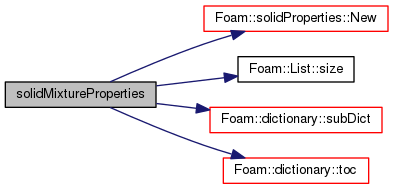Here is the caller graph for this function: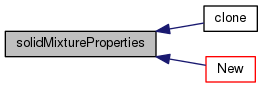solidMixtureProperties ( const solidMixtureProperties & lm )

Construct copy.

Definition at line 45 of file solidMixtureProperties.C.

References forAll, and solidMixtureProperties::New().

Here is the call graph for this function: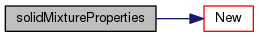virtual ~solidMixtureProperties ( )
inlinevirtual

Destructor.

Definition at line 106 of file solidMixtureProperties.H.

References solidMixtureProperties::New().

Here is the call graph for this function: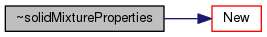## Member Function Documentation

 virtual autoPtr clone ( ) const
inlinevirtual

Construct and return a clone.

Definition at line 96 of file solidMixtureProperties.H.

Here is the call graph for this function: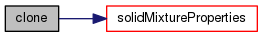Foam::autoPtr< Foam::solidMixtureProperties > New ( const dictionary & thermophysicalProperties )
static

Select construct from dictionary.

Definition at line 62 of file solidMixtureProperties.C.

Here is the call graph for this function: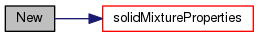Here is the caller graph for this function: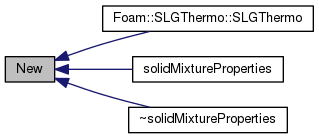const List& components ( ) const
inline

Return the solidProperties names.

Definition at line 119 of file solidMixtureProperties.H.

 const PtrList& properties ( ) const
inline

Return the solidProperties properties.

Definition at line 125 of file solidMixtureProperties.H.

Here is the caller graph for this function: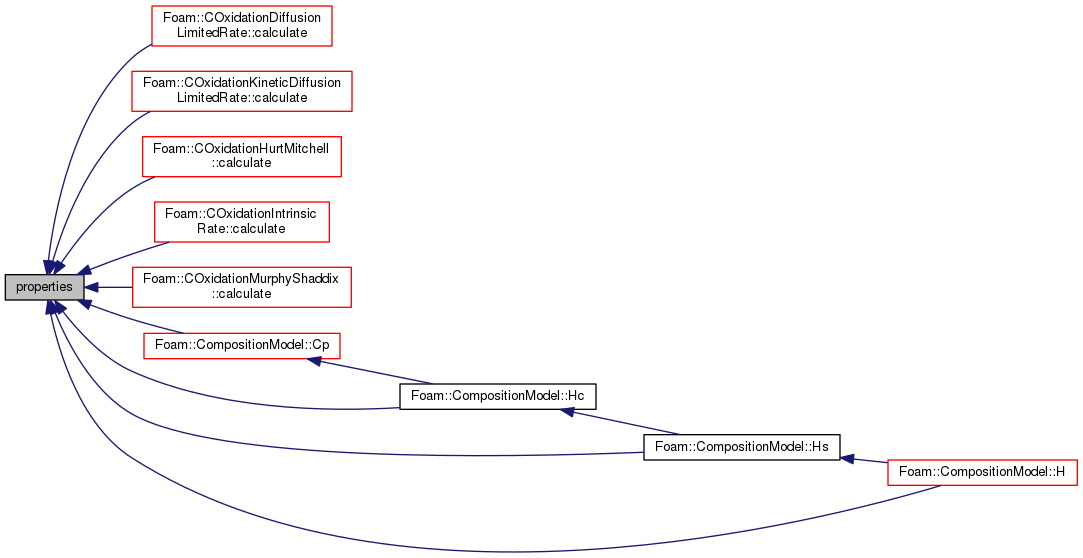label size ( ) const
inline

Return the number of solids in the mixture.

Definition at line 131 of file solidMixtureProperties.H.

Here is the call graph for this function: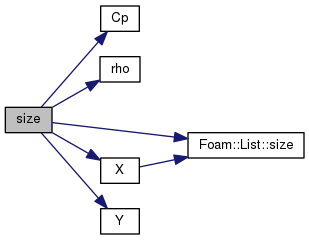scalarField Y ( const scalarField & X ) const

Returns the mass fractions, given mole fractions.

Referenced by solidMixtureProperties::size().

Here is the caller graph for this function: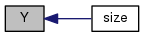Foam::scalarField X ( const scalarField & Y ) const

Returns the mole fractions, given mass fractions.

Definition at line 75 of file solidMixtureProperties.C.

References forAll, and List< T >::size().

Referenced by solidMixtureProperties::size().

Here is the call graph for this function: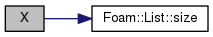Here is the caller graph for this function: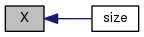Foam::scalar rho ( const scalarField & X ) const

Calculate the mixture density [kg/m^3] as a function of.

volume fractions

Definition at line 90 of file solidMixtureProperties.C.

References forAll.

Referenced by solidMixtureProperties::size().

Here is the caller graph for this function: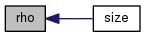Foam::scalar Cp ( const scalarField & Y ) const

Calculate the mixture heat capacity [J/(kg K)] as a function.

of mass fractions

Definition at line 101 of file solidMixtureProperties.C.

References forAll.

Referenced by solidMixtureProperties::size().

Here is the caller graph for this function: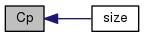The documentation for this class was generated from the following files: